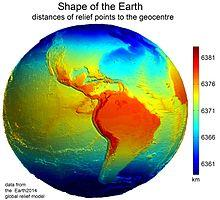# Average speed

What is the average speed you have to move the way around the world in 80 days? (Path along the equator, round to km/h).

Result

v =  21 km/h

#### Solution:

$t = 80 \cdot \ 24 = 1920 \ \\ r = 6371 \ \\ s = 2 \pi \cdot \ r = 2 \cdot \ 3.1416 \cdot \ 6371 \doteq 40030.1736 \ \\ v = s/t = 40030.1736/1920 \doteq 20.849 = 21 \ \text { km/h }$Our examples were largely sent or created by pupils and students themselves. Therefore, we would be pleased if you could send us any errors you found, spelling mistakes, or rephasing the example. Thank you!

Leave us a comment of this math problem and its solution (i.e. if it is still somewhat unclear...):Be the first to comment!#### Following knowledge from mathematics are needed to solve this word math problem:

Do you want to convert length units?

## Next similar math problems:

1. Rotation of the EarthCalculate the circumferential speed of the Earth's surface at a latitude of 61°​​. Consider a globe with a radius of 6378 km.
2. BallsPing pong balls have a diameter of approximately 5.1 cm. They are sold in boxes of 10 pieces: each box has the shape of a cuboid with a square base. The balls touch the walls of the box. Calculate what portion of the internal volume of the box is filled.
3. SphereThe surface of the sphere is 12100 cm2, and the weight is 136 kg. What is its density?
4. Spherical capFrom the sphere of radius 13 was truncated spherical cap. Its height is 6. What part of the volume is spherical cap from whole sphere?
5. Sphere A2VSurface of the sphere is 241 mm2. What is its volume?
6. Cube in a sphereThe cube is inscribed in a sphere with volume 7253 cm3. Determine the length of the edges of a cube.
7. CubesOne cube is inscribed sphere and the other one described. Calculate difference of volumes of cubes, if the difference of surfaces in 257 mm2.
8. MO SK/CZ Z9–I–3John had the ball that rolled into the pool and it swam in the water. Its highest point was 2 cm above the surface. Diameter of circle that marked the water level on the surface of the ball was 8 cm. Determine the diameter of John ball.
9. Earth parallelEarth's radius is 6370 km long. Calculate the length parallel of latitude 50°.
10. Sphere slicesCalculate volume and surface of a sphere, if the radii of parallel cuts r1=31 cm, r2=92 cm and its distance v=25 cm.
11. Hollow sphereCalculate the weight of a hollow tungsten sphere (density 19.3 g/cm3), if the inner diameter is 14 cm and wall thickness is 3 mm.
12. BallsThree metal balls with volumes V1=71 cm3 V2=78 cm3 and V3=64 cm3 melted into one ball. Determine it's surface area.
13. Cube in ballCube is inscribed into sphere of radius 241 cm. How many percent is the volume of cube of the volume of sphere?
14. Sphere in coneA sphere of radius 3 cm desribe cone with minimum volume. Determine cone dimensions.
15. Two ballsTwo balls, one 8cm in radius and the other 6cm in radius, are placed in a cylindrical plastic container 10cm in radius. Find the volume of water necessary to cover them.
16. Hollow sphereSteel hollow sphere floats on the water plunged into half its volume. Determine the outer radius of the sphere and wall thickness, if you know that the weight of the sphere is 0.5 kg and density of steel is 7850 kg/m3
17. Spherical segmentSpherical segment with height h=7 has a volume V=198. Calculate the radius of the sphere of which is cut this segment.